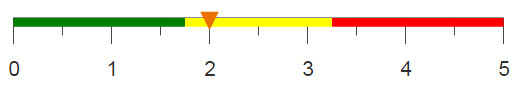# Creating pairs without duplication

You can create a list of every possible combination of two lists of items by performing a link and expand. However, with this method, you will get duplicate pairs, where the items are simply listed in a different order (i.e., reciprocals). Using computed columns and g_functions, you can create a list of pairs without any reciprocals.

## Difficulty## Objective

You want to compute the affinity of every pair of items bought together. To complete this analysis, you need to create the pairs within each basket from a transaction table. However, this table is already large, so performing a link and expand to create the pairs will create an even larger worksheet, as well as duplicate pairs. To avoid these inefficiencies, you should only expand the table as much as necessary to allow for the creation of all pairs of items without creating duplicates.

## Solution

```<block name="expand_prep">
<sel value="year(trans_date)=2016 & month(trans_date)=03"/>
<sel value="g_first1(transid sku;;)"/>
<willbe name="order" value="g_cumcnt(transid;;)"/>
<willbe name="num" value="g_ucnt(transid;;sku)"/>
<willbe name="expand" value="num-order"/>
</block>
<base table="pub.doc.retail.altseg.sales_detail_transid"/>
<insert block="expand_prep"/>
<sel value="expand" expand="1"/>
<willbe name="linker" value="order+ii_(1)"/>
<link table2="pub.doc.retail.altseg.sales_detail_transid"
col="transid,linker" col2="transid,order">
<insert block="expand_prep"/>
<colord cols="transid,sku,order"/>
</link>
<colord cols="transid,sku,c1"/>```

## Discussion

Before beginning any expansions, a selection is done on the table in order to limit the results to a specific time period. This will help prevent the table from becoming unnecessarily large. Additionally, only the first instance of each transaction/SKU is selected because if a specific item is bought twice in one transaction, you don't need to compare it to itself.

Now that the table is reduced to only the necessary data points, you can start creating the preparatory columns for the expansion. First, you need a column that specifies the order of products within each transaction. This can be done by performing a cumulative count for each transaction. The order of the products is important because each product is expanded in order to accommodate the products that appear after it, therefore a definitive order is needed. Then, you need a column that indicates how many products are in each transaction, which is calculated with g_ucnt(G;S;X). By subtracting the order of each product from the number of products contained in the transaction, you can determine how many times to expand each row.

Each item in the transaction will be expanded to accommodate the number of unique products that appear thereafter in the same transaction. Using this method, the first item will be expanded once for every item in the transaction excluding itself. The next item will be expanded one time less than the first and so on, until the last item is actually not expanded at all, but rather removed from the table, as it will have appeared in every prior pair.

Once the table is expanded, it is linked to itself using the linker column and the order column. The column, linker, is created by adding the enumeration of each SKU in each transaction to that SKU's order. For example, SKU 112741 in transaction -2081370256 is expanded three times, and it's the first SKU listed in the transaction. Therefore, in the linker column, the first instance of the SKU will have a value of 2, (1+1), the second instance will have a value of 3, (1+2), and the third instance will have a value of 4, (1+3). Linking this column to the order column from the same table, will create the unique pairs of items.

Note: This method only works if the order of the items in each pair does not matter. For example, if you need to compare apples and oranges but it doesn't matter if you're comparing apples to oranges or oranges to apples, this methodology will work. However, if you need to know how apples compare to oranges and how oranges compare to apples, you should perform a link and expand which will give you these reciprocal pairs.

## Common errors

There was a problem getting a worksheet for a link (this can be caused by the worksheet being too large). The trapped error was: Too much data to get from table at subprocesses; hide some columns or select fewer rows
If the table that you are working with is larger than 10,000,000 rows after the expansion, it will be too large to link to itself due to worksheet size limitations. In order to avoid this error, you can make further selections on your original table in order to decrease the worksheet size.

## Further reading

If you would like to learn more about the functions and operations discussed in this recipe, click on the links below:

<sel>

<link>

g_cumcnt(G;S;O)

g_ucnt(G;S;X)